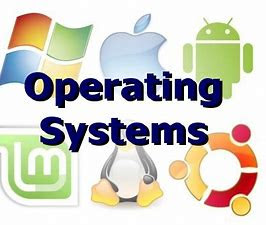# C-Program of C- Look Disk scheduling Algorithms in operating system (OS).

What is C- Look disk scheduling:-

As LOOK is similar to SCAN algorithm, in similar way, CLOOK is similar to CSCAN disk scheduling algorithm. In CLOOK, the disk arm in spite of going to the end goes only to the last request to be serviced in front of the head and then from there goes to the other end’s last request. Thus, it also prevents the extra delay which occurred due to unnecessary traversal to the end of the disk.

Example:- Given the following queue — 95, 180, 34, 119, 11, 123, 62, 64 with the Read-write head initially at the track 50 and the tail track being at 199.Head movement is towards low values.

1. Starvation is avoided in C-LOOK.
2. Low variance is provided in waiting time and response time.

1. An overhead to find the end request.

Code:-

#include<stdio.h>
#include<stdlib.h>
int main()
{
printf(“Enter the number of Requestsn”);
scanf(“%d”,&n);
printf(“Enter the Requests sequencen”);
for(i=0;i<n;i++)
scanf(“%d”,&RQ[i]);
printf(“Enter initial head positionn”);
scanf(“%d”,&initial);
printf(“Enter total disk sizen”);
scanf(“%d”,&size);
printf(“Enter the head movement direction for high 1 and for low 0n”);
scanf(“%d”,&move);
// logic for C-look disk scheduling
/*logic for sort the request array */
for(i=0;i<n;i++)
{
for( j=0;j<ni1;j++)
{
if(RQ[j]>RQ[j+1])
{
int temp;
temp=RQ[j];
RQ[j]=RQ[j+1];
RQ[j+1]=temp;
}

}
}

int index;
for(i=0;i<n;i++)
{
if(initial<RQ[i])
{
index=i;
break;
}
}
// if movement is towards high value
if(move==1)
{
for(i=index;i<n;i++)
{
initial=RQ[i];
}
for( i=0;i<index;i++)
{
initial=RQ[i];
}
}
// if movement is towards low value
else
{
for(i=index1;i>=0;i–)
{
initial=RQ[i];
}
for(i=n1;i>=index;i–)
{
initial=RQ[i];
}
}
return 0;
}

Output:-

Enter the number of Request
8
Enter the Requests Sequence
95 180 34 119 11 123 62 64
Enter initial head position
50
Enter the head movement direction for high 1 and for low 0
1
Total head movement is 322Must visit:-

Recommended Post:

Hackerearth Problems:-

Data structure:-

Key points:-

MCQs:-